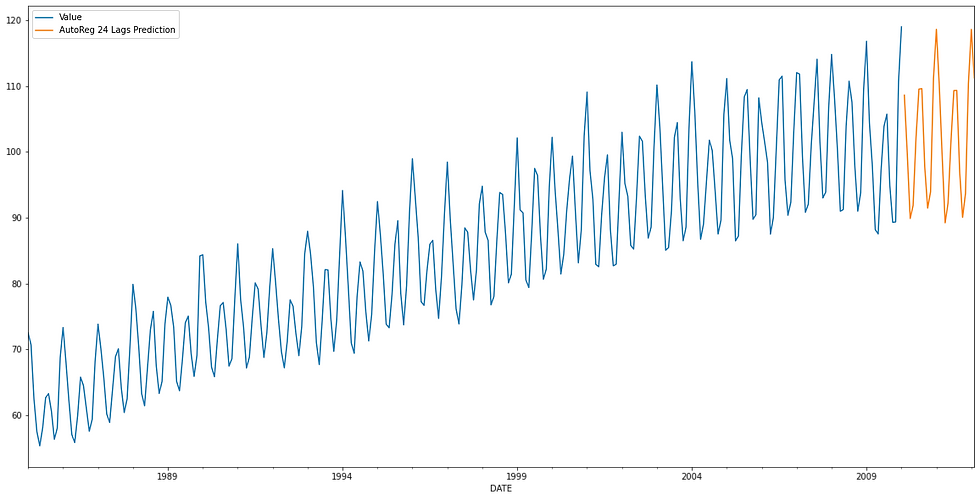top of page
• onur baltacı

# Analysis and Forecast of Electricity Consumption

Güncelleme tarihi: 21 Şub

Hello, in this blog post, we will try to analyze the electricity consumption and after that we will do a prediction using an Autoregressive model. When we are working with time series data we need to know that there can be various factors effecting our values. We can't completely trust in predictions if we are not taking all the factors that can effect our values. You can get the data set from: Data set link.

`data = pd.read_csv("Course Data Set.csv",index_col = "DATE", parse_dates = True)`

Let's see the first 5 entry.

`data.head()`And see numerical summary of our data.

`data.describe()`Now we will increase the figure size by the following code. This can only effect if we add plt.show() after the plots since we will be plotting with pandas.

`matplotlib.rc('figure', figsize=(20, 10))`

We can visualize our data and see how it looks now.

```data.plot()
plt.show()```It looks like there is seasonality in our data. We can see an increase starting from the beginning and ends around 2004. After that our electric consumption values seems stable. I am not going to include all that time in our model so we are going to write a code which will take the time between 1985 and 2010.

```start_date = "1985-01-01"
end_date = "2010-01-01"
mask = (data.index >= start_date) & (data.index <= end_date)

```data.plot()
plt.show()```Seems nice. Let's plot the yearly averages. We can expect to have smoother graph. We will use rolling for this and our window value will be 12 in order to have yearly values.

```yearlydata = data.rolling(window = 12).mean()
yearlydata.plot()
plt.show()```Let's add some lines to our graph in order to see seasonality better. We will add lines to each year.

```xcoords = ["1986-01-01","1987-01-01","1988-01-01","1989-01-01","1990-01-01","1991-01-01","1992-01-01","1993-01-01","1994-01-01","1995-01-01","1996-01-01","1997-01-01","1998-01-01","1999-01-01","2000-01-01","2001-01-01","2002-01-01","2003-01-01","2004-01-01","2005-01-01","2006-01-01","2007-01-01","2008-01-01","2009-01-01"]
data.plot()
for xc in xcoords:
plt.axvline(x=xc, color = "black",linestyle = "--")
plt.show()```Now we can see the seasonality better. Lets also use seasonal decomposition.

```decompose = seasonal_decompose(data["Value"],model="additive")
decompose.plot()
plt.show()```Now we are going to do Dickey-Fuller Test for testing the stationarity in our data. We will define a function for that and our function will let us know that the stationarity statue.

```def adf_test(series):
labels = ["ADF Test Statistic", "p value", "# of lags", "# of observations"]
out = pd.Series(result[0:4],index = labels)
print(out.to_string)
if result <= 0.05:
print("Reject the null hypothesis")
print("Data has no unit root and stationary")
else:
print("Fail to reject null hypothesis")
print("Data has unit root and non-stationary")

Output is:

p value 0.301107

# of lags 15.000000

# of observations 285.000000

dtype: float64>

Fail to reject null hypothesis

Data has unit root and non-stationary

Now we can train our autoregressive model. Statsmodels is updated and now we need to pass lag value in for training autoregressive model. So for taking the best performing one in terms of aic score, we will create a for loop which will try the lags between 1 and 30 and add the aic scores of models into our list.

```aic_scores = []
for index in range(1,30):
model = AutoReg(data["Value"],lags = index)
model_fitted = model.fit()
aic_scores.append(model_fitted.aic)```

Seems nice. Let's see the best performing lag.

`aic_scores.index(min(aic_scores))`

Output is 23. It means best performing lag value is 24 (index starts with 0 in Python list). Let's see the aic score of it.

`aic_scores`

It is 1.82. Let's build our model again with 24 lags and get the summary of it.

```model = AutoReg(data["Value"],lags = 24)
model_fitted = model.fit()
print(model_fitted.summary())```Ok, we can see the coefficients and much more from our summary table. Let's do the forecast. We will be predicting 2 years.

`fcast = model_fitted.predict(start = len(data),end= len(data) + 24)`

And at the final step, we are going to visualize it.

```fcast = fcast.rename("AutoReg 24 Lags Prediction")
data.plot(legend=True)
fcast.plot(legend=True)
plt.show()```